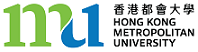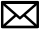Skip to Main Content# Mathematics & Statistics 數學及統計學: Books 書籍

Welcome to our library guides! Here you can find useful information such as how to search for journal articles, printed or electronic books, etc relating to this subject. 歡迎使用本指南！你可以在這裡學到如何尋找與這科目有關的期刊文章、印刷本書籍和電子書等參考資料。

## Online Tutorial 網上教室

The video below shows you how to search for Print Books and E-Books in LibSearch.

 收看中文版影片 收看中文版影片

## Search library resources by keywords 建議關鍵字

This guide is about Mathematics & Statistics, which covers the following subject areas. You may use these keywords to search for related resources in LibSearch.

 Applied Mathematics Algebra Algorithms Business Mathematics Calculus Combinatorics Computer Algorithms Decision Analysis Decision Making – Mathematical Models Differential Equations Economics, Mathematical Finance -- Mathematical Models Geometry Linear Models (Statistics) Logic, Symbolic and Mathematical Mathematical Models Mathematical Sciences Mathematical Statistics Number Theory Probabilities Set Theory Statistical Decision Statistical Methods Statistical Modelling Trigonometry

## Call Number relating to this subject 相關書籍的索書號

Books on Mathematics & Statistics can be found on the shelves according to the following classification codes:

Classification Codes 分類號碼 Classification Outline 包含範圍
HA 1-4737 Statistics
QA 1 – 939 Mathematics
QA 1 – 43 General
QA 47 – 59 Tables
QA 71 – 90 Instruments and Machines
QA 75 – 76.95 Calculating Machines
QA 150 – 272.5 Algebra
QA 273 – 280 Probabilities, Mathematical Statistics
QA 299.6 – 433 Analysis
QA 440 – 699 Geometry, Trigonometry, Topology
QA 801 – 939 Analytic Mechanics

## How to reserve or renew? 如何預約或續借？2768 6777libwww@hkmu.edu.hk